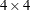# The PLAN Procedure

### Example 72.4 A Latin Square Design

All of the preceding examples involve designs with completely nested block structures, for which PROC PLAN was especially designed. However, by appropriate coordination of its facilities, PROC PLAN can accommodate a much wider class of designs. A Latin square design is based on experimental units that have a row-and-column block structure. The following statements use the CYCLIC option in the TREATMENTS statement to generate a simpleLatin square. The RANDOM option in the OUTPUT statement randomizes the generated Latin square by randomly permuting the row, column, and treatment values independently. This example also uses factor-value-settings in the OUTPUT statement.

title 'Latin Square Design';
proc plan seed=37430;
factors Row=4 ordered Col=4 ordered / noprint;
treatments Tmt=4 cyclic;
output out=LatinSquare
Row cvals=('Day 1' 'Day 2' 'Day 3' 'Day 4') random
Col cvals=('Lab 1' 'Lab 2' 'Lab 3' 'Lab 4') random
Tmt nvals=(      0     100     250     450) random;
quit;

proc sort data=LatinSquare out=LatinSquare;
by Row Col;
run;
proc transpose data= LatinSquare(rename=(Col=_NAME_))
out =tLatinSquare(drop=_NAME_);
by Row;
var Tmt;
run;
proc print data=tLatinSquare noobs;
run;


The preceding statements produce Output 72.4.1.

Output 72.4.1: A Randomized Latin Square Design

 Latin Square Design

Row Lab_1 Lab_2 Lab_3 Lab_4
Day 1 0 250 100 450
Day 2 250 450 0 100
Day 3 100 0 450 250
Day 4 450 100 250 0

You can use the PLAN procedure to randomize Latin squares from any transformation sets. See Kempthorne (1952) for definitions of transformation sets. In particular, the following DATA step and PROC PLAN statements demonstrate how to randomize a Latin square from the second transformation set defined in Kempthorne (1952). The following DATA step creates aLatin square from the transformation set 2:

data Unrandomized;
do Row = 1 to 4;
do Col = 1 to 4;
input Tmt @@;
output;
end;
end;
datalines;
1 2 3 4
2 1 4 3
3 4 1 2
4 3 2 1
;


The following PROC PLAN statements permute the rows and columns and randomly assign treatment levels.

proc plan seed=37430;
factors Row = 4;
output data=  Unrandomized  out=Randomized1;
run;
factors Col = 4;
output data=  Randomized1   out=Randomized2;
run;
factors Tmt = 4;
output data=  Randomized2   out=Randomized3;
run;

proc sort data=Randomized3;
by Row Col;
run;
proc transpose data= Randomized3 out =tLatinSquare2(drop=_NAME_);
by Row;
var Tmt;
run;
proc print data=tLatinSquare2 noobs;
run;


Output 72.4.2: A Randomized Latin Square from a Different Transformation Set

The PLAN Procedure

Factor Select Levels Order
Row 4 4 Random

Row
1 3 2 4

Factor Select Levels Order
Col 4 4 Random

Col
3 2 4 1

Factor Select Levels Order
Tmt 4 4 Random

Tmt
4 3 2 1

Row COL1 COL2 COL3 COL4
1 1 3 4 2
2 3 1 2 4
3 2 4 3 1
4 4 2 1 3